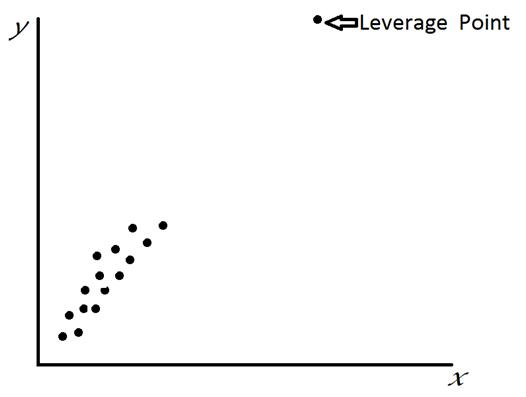# Leverage Points - Meaning & Definition

## What is Leverage Points?

Leverage Points is an observation which usually lies away from the rest of the data on the x-y plane but lies close to the regression line when extended. This regression line is determined by the data other than the leverage point.

A point is determined as a leverage point based on the location of the point on the x-space and hence remote points impart more on the parameters of the model. To identify a leverage point, a hat matrix:

H= X(X’X)-1X’

is used.If a data point i, is moved up or moved down, the corresponding fitted value yi’moves proportionally to the change in yi. The proportionality constant used is called Leverage which is denoted by hi.Hence each data point has a leverage value. The entries on the diagonal for the matrix H determines the measure for how far the ith observation lays from the center of the x-space and hence larger values correspond to potential influential points. Usually the average of this diagonal for the hat matrix is the average of this diagonal for the hat matrix is p/n and hence for elements hii , if the value exceeds 2p/n, then it is a leverage point.

Hence, this concludes the definition of Leverage Points along with its overview.

This article has been researched & authored by the Business Concepts Team. It has been reviewed & published by the MBA Skool Team. The content on MBA Skool has been created for educational & academic purpose only.

Browse the definition and meaning of more similar terms. The Management Dictionary covers over 2000 business concepts from 5 categories.